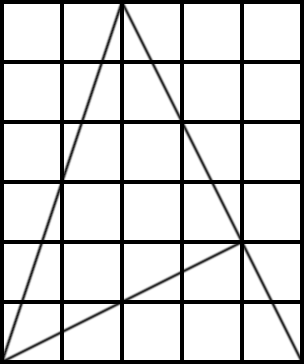# Proof Without WordsIn the January 2001 issue of the College Mathematics Journal, University of Calgary mathematician Jonathan Schaer offers this simple proof that arctan 1 + arctan 2 + arctan 3 = π. The sum of the angles of this large triangle is 180°. And the diagram shows that its lower left angle is arctan 3, its lower right angle is arctan 2, and its top angle is part of an isosceles right triangle. So arctan 1 + arctan 2 + arctan 3 = 180°, or π radians. “No words, even no symbols!”

(“Miscellanea,” College Mathematics Journal 32:1, 68-71.)